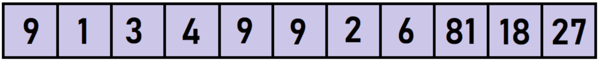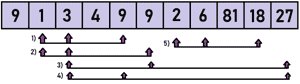Related Tags

python
algorithm
communitycreator

# How to find all ordered 3-item geometric progressions in a list

Dhruv Sharma

### Understanding the problem

Let's say the given common ratio is 3 and the given list is:For the given list, the possible geometric progression of size 3 with an index such that A*1 < A*2 < A*3 are:

( (index , number) , (index , number) , (index , number) )

1. ( (1,1) , (2,3) , (4,9) )
2. ( (1,1) , (2,3) , (5,9) )
3. ( (2,3) , (4,9) , (10,27) )
4. ( (2,3) , (5,9) , (10,27) )
5. ( (6,2) , (7,6) , (9,18) )### Explanation

1) mainl and r variables contains the list and the common ratio.

2) left and right dictionary are initialized to keeps track of how many times each number occurs to the left and right of each index in mainl .

3) While iterating through mainl, we initiate each element in left and right. Values in left elements are initially 0 and right elements are total number of occurrence of each element in mainl .

1. For this example, left and right are:
1. right = {9: 3, 1: 1, 3: 1, 4: 1, 2: 1, 6: 1, 81: 1, 18: 1, 27: 1}
2. left = {9: 0, 1: 0, 3: 0, 4: 0, 2: 0, 6: 0, 81: 0, 18: 0, 27: 0}

4) Count variable keep track of number of geometric progressions found.

5) While iterating through mainl again , for each element num :

1. We initiate 2 variables high and low with 0.
1. We subtract 1 from right[num] as the element cannot occur to the right of itself.
• For first iteration (index = 0) left and right becomes:
1. right = {9: 2, 1: 1, 3: 1, 4: 1, 2: 1, 6: 1, 81: 1, 18: 1, 27: 1}
2. left = {9: 0, 1: 0, 3: 0, 4: 0, 2: 0, 6: 0, 81: 0, 18: 0, 27: 0}
• For second iteration (index = 1) left and right becomes:
1. right = {9: 2, 1: 0, 3: 1, 4: 1, 2: 1, 6: 1, 81: 1, 18: 1, 27: 1}
2. left = {9: 1, 1: 0, 3: 0, 4: 0, 2: 0, 6: 0, 81: 0, 18: 0, 27: 0}
• For third iteration (index = 2) left and right becomes:
1. right = {9: 2, 1: 0, 3: 0, 4: 1, 2: 1, 6: 1, 81: 1, 18: 1, 27: 1}
2. left = {9: 1, 1: 1, 3: 0, 4: 0, 2: 0, 6: 0, 81: 0, 18: 0, 27: 0}
2. We update high and low with right[num*r] and left[num/r] as GP can be written as a/n , a , a*n.
• For third iteration (index = 0) high and low are:
1. High = right[9*3] = 2
2. Low = left[9/3] = 0
• For third iteration (index = 1) high and low are:
1. High = right[1*3] = 1
2. Low = left[1/3] = 0
• For third iteration (index = 2) high and low are:
1. High = right[3*3] = 2
2. Low = left[3/3] = 1
3. We add high*low to count as high*low is equal to number of geometric progression with that index as middle of the 3 element.
• For first element (index=0):
1. count += high * low = 2 * 0 = 0
• For first element (index=1):-
1. count += high * low = 1 * 0 = 0
• For first element (index=2):-
1. count += high * low = 2 * 1 = 2
4. We add 1 to left[num] as the current element will occur to left of every next element.
• After iterating through first element (index=0):
1. left = {9: 1, 1: 0, 3: 0, 4: 0, 2: 0, 6: 0, 81: 0, 18: 0, 27: 0}
2. right = {9: 2, 1: 1, 3: 1, 4: 1, 2: 1, 6: 1, 81: 1, 18: 1, 27: 1}
• After iterating through first element (index=0):
1. left = {9: 1, 1: 1, 3: 0, 4: 0, 2: 0, 6: 0, 81: 0, 18: 0, 27: 0}
2. right = {9: 2, 1: 0, 3: 1, 4: 1, 2: 1, 6: 1, 81: 1, 18: 1, 27: 1}
• After iterating through first element (index=0):
1. left = {9: 1, 1: 1, 3: 1, 4: 0, 2: 0, 6: 0, 81: 0, 18: 0, 27: 0}
2. right = {9: 2, 1: 0, 3: 0, 4: 1, 2: 1, 6: 1, 81: 1, 18: 1, 27: 1}

6) We print count .

RELATED TAGS

python
algorithm
communitycreator

CONTRIBUTOR

Dhruv Sharma
RELATED COURSES

View all Courses

Keep Exploring

Learn in-demand tech skills in half the time